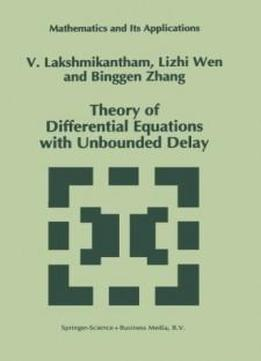# Theory Of Differential Equations With Unbounded Delay (mathematics And Its Applications) by V. Lakshmikantham / 2013 / English / PDF

Because the theory of equations with delay terms occurs in a variety of contexts, it is important to provide a framework, whenever possible, to handle as many cases as possible simultaneously so as to bring out a better insight and understanding of the subtle differences of the various equations with delays. Furthermore, such a unified theory would avoid duplication and expose open questions that are significant for future research.

Because the theory of equations with delay terms occurs in a variety of contexts, it is important to provide a framework, whenever possible, to handle as many cases as possible simultaneously so as to bring out a better insight and understanding of the subtle differences of the various equations with delays. Furthermore, such a unified theory would avoid duplication and expose open questions that are significant for future research. It is in this spirit that the authors view the importance of their monograph, which presents a systematic and unified theory of recent developments of equations with unbounded delay, describes the current state of the theory showing the essential unity achieved, and provides a general structure applicable to a variety of problems.

It is in this spirit that the authors view the importance of their monograph, which presents a systematic and unified theory of recent developments of equations with unbounded delay, describes the current state of the theory showing the essential unity achieved, and provides a general structure applicable to a variety of problems. It is the first book that:

It is the first book that: (i) presents a unified framework to investigate the basic existence theory for a variety of equations with delay;

(i) presents a unified framework to investigate the basic existence theory for a variety of equations with delay; (ii) treats the classification of equations with memory precisely so as to bring out the subtle differences between them;

(ii) treats the classification of equations with memory precisely so as to bring out the subtle differences between them; (iii) develops a systematic study of stability theory in terms of two different measures which includes several known concepts; and

(iii) develops a systematic study of stability theory in terms of two different measures which includes several known concepts; and (iv) exhibits the advantages of employing Lyapunov functions on product spaces as well as the method of perturbing Lyapunov functions.

(iv) exhibits the advantages of employing Lyapunov functions on product spaces as well as the method of perturbing Lyapunov functions. This book will be of value to researchers and advanced graduate students in mathematics, electrical engineering and biomathematics.

This book will be of value to researchers and advanced graduate students in mathematics, electrical engineering and biomathematics.

views: 688